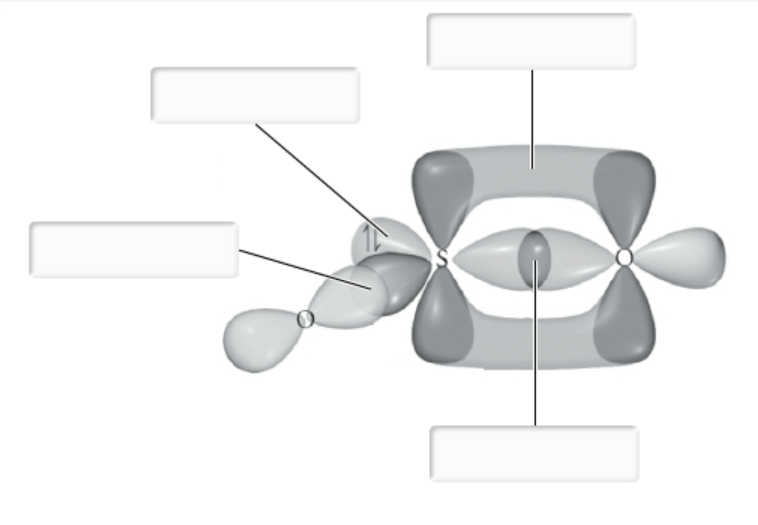# Problem: Label all bonds in SO2.The hybridization of the S atom in SO2 is sp2.δ: S(sp2) - O(p)Lone pair in sp2 orbitalδ: S (p) - O(sp2)π: S(p) - O(p)π: S(p) - O(sp2)Lone pair in p orbitalδ: S(p) - O(p)π: S(sp2) - O(p)

###### FREE Expert Solution

We are asked to label all the bonds in SO2.

Step 1: Sulfur (EN = 2.5) is less electronegative than oxygen (EN = 3.5) so sulfur is the central atom

Step 2: The total number of valence electrons present in SO2 is:

Group             Valence Electrons

S       6A                   1 × 6 e6 e

O       6A                   2 × 6 e12 e

Total: 18 valence e

Step 3: Oxygen prefers to form 2 bonds. The Lewis structure is:

86% (223 ratings)###### Problem Details

Label all bonds in SO2.

The hybridization of the S atom in SO2 is sp2.δ: S(sp2) - O(p)

Lone pair in sp2 orbital

δ: S (p) - O(sp2)

π: S(p) - O(p)

π: S(p) - O(sp2)

Lone pair in p orbital

δ: S(p) - O(p)

π: S(sp2) - O(p)